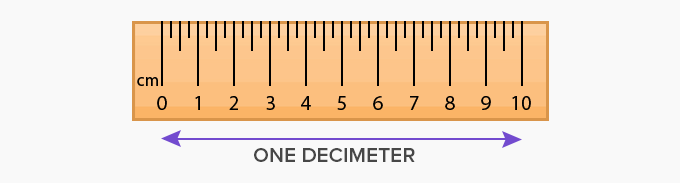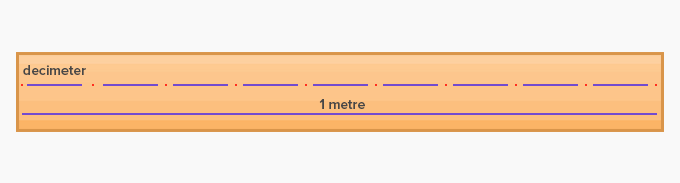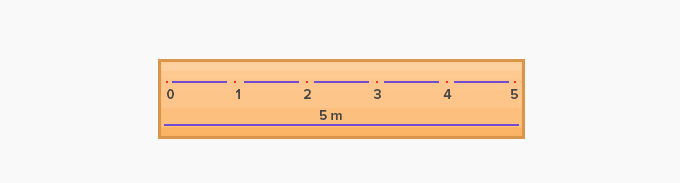# Decimeter - Definition with Examples

The Complete K-5 Math Learning Program Built for Your Child

• 40 Million Kids

Loved by kids and parent worldwide

• 50,000 Schools

Trusted by teachers across schools

• Comprehensive Curriculum

Aligned to Common Core

## What is Decimeter?

A decimeter is a unit of length in the metric system. The term “Deci” means one-tenth, and therefore decimetre means one-tenth of a meter. Since a meter is made up of 100 cm, one-tenth of 100 cm is 10 cm. Thus one decimeter measures 10 cm.The symbol used to write decimeter is dm.Scale showing 1 meter divided into 10 parts. Each part measures 1 decimeter.

Few conversions for decimeter are as follows:

• 1 dm = 10 cm

• 1 dm = 100 mm

• 1 dm = 0.1 m

The type of problems for decimeter shall mostly involve the conversion of units. Following are some examples:

1.    How many decimeters do 5 meters include?

Solution:1 m = 100 cm

Therefore, 5 m = 100 × 5 = 500 cm

Since, 1 dm =  0.1  m

Therefore, 500 cm will be 500 × 110

Hence, 5 m = 50 dm

2.   Convert  250 dm into centimetres

Solution:

1 dm = 10 cm

Therefore, 250 dm = 250 × 10

= 2500 cm

The commonly used units of measurement are millimeter, centimeter, meter, and kilometer. A decimeter is not as widely used, but is an important unit. In real life, we rarely find measurements written in decimetre. Since one meter is not a very long length, it is easier to use 0.1 m or 0.5 m when the length is shorter than a meter.

 Fun Facts Decimeter is a unit that is larger than millimeter and centimeter. In the increasing order of units, decimeter is in the third position and further the units increase up to kilometers.

Won Numerous Awards & Honors